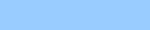*   Techniques of integration Solution to first order differential equations by separation of variablesFormation of a differential equation Solution to first order differential equations by means of an integrating factor Linear motion under a variable force *  Calculus:summary *  Linear motion of a body of variable mass *  Simple harmonic motion